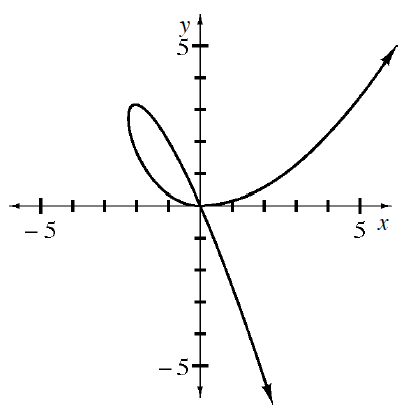### Home > APCALC > Chapter 6 > Lesson 6.4.1 > Problem6-124

6-124.

The graph of $x^3 = 5xy + 2y^2$ is shown below.

1. How many tangent lines exist at $x = -2$?

How many coordinate points does this graph have at
$x = −2$?

2. Write the equations of the tangent lines at $x = -2$.

Write two tangent line equations in point-slope form.
Because you used implicit differentiation, your derivative might have both $x$- and $y$-values.
That means you will need $\left(x, y\right)$ coordinate points to evaluate.